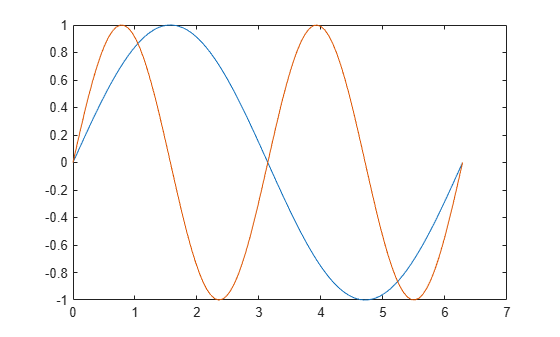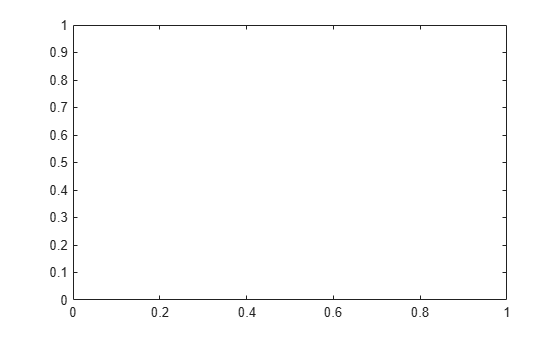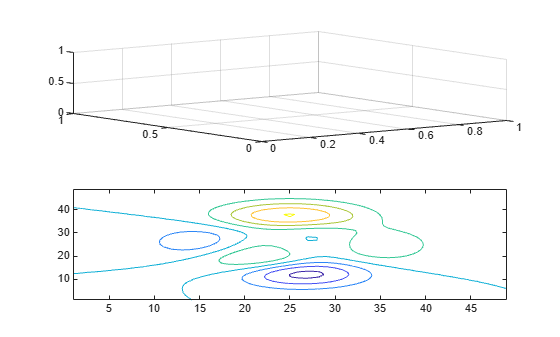cla

Description

example

cla deletes all graphics objects that have visible handles from the current axes. The handle is visible if the HandleVisibility property of the object is set to 'on'. The next plot added to the axes uses the first color and line style based on the ColorOrder and LineStyleOrder properties of the axes. If axes do not exist, then this command creates one.

example

cla(ax) deletes graphics objects from the axes, polar axes, or geographic axes specified by ax instead of the current axes.

example

cla reset deletes graphics objects from the current axes regardless of their handle visibility. It also resets axes properties to their default values, except for the Position and Units properties.

example

cla(ax,'reset') resets properties for the specified axes.

Examples

collapse all

Plot two sine waves. Then, clear the line plots from the axes.

x = linspace(0,2*pi);
y1 = sin(x);
plot(x,y1)

hold on
y2 = sin(2*x);
plot(x,y2)clacla clears the line plots and resets the ColorIndex and LineStyleIndex properties of the axes to 1. Subsequent plots start from the beginning of the color order and line style order. For example, plot another sine wave.

y3 = sin(3*x);
plot(x,y3)
hold offStarting in R2019b, you can display a tiling of plots using the tiledlayout and nexttile functions. Call the tiledlayout function to create a 2-by-1 tiled chart layout. Call the nexttile function to create the axes objects ax1 and ax2. Add plots to both axes.

tiledlayout(2,1)
ax1 = nexttile;
surf(ax1,peaks)

ax2 = nexttile;
contour(ax2,peaks)Clear the surface plot from the upper axes by specifying ax1 as an input argument to cla.

cla(ax1)Now, reset all properties for the axes, including the camera properties that control the view, by using the optional input argument 'reset'.

cla(ax1,'reset')Create a line plot and set the axis limits.

x = linspace(0,2*pi);
y = sin(x);
plot(x,y)
axis([0 5 -2 2])Clear the line plot from the axes and reset all the axes properties to their default values. cla reset resets all properties of the current axes, except for the Position and Units properties.

cla resetInput Arguments

collapse all

Target axes, specified as an Axes object, a PolarAxes object, or a GeographicAxes object. Use ax to clear specific axes, instead of the current axes.

Algorithms

The cla command resets the ColorOrderIndex and LineStyleOrderIndex properties of the current axes to 1.